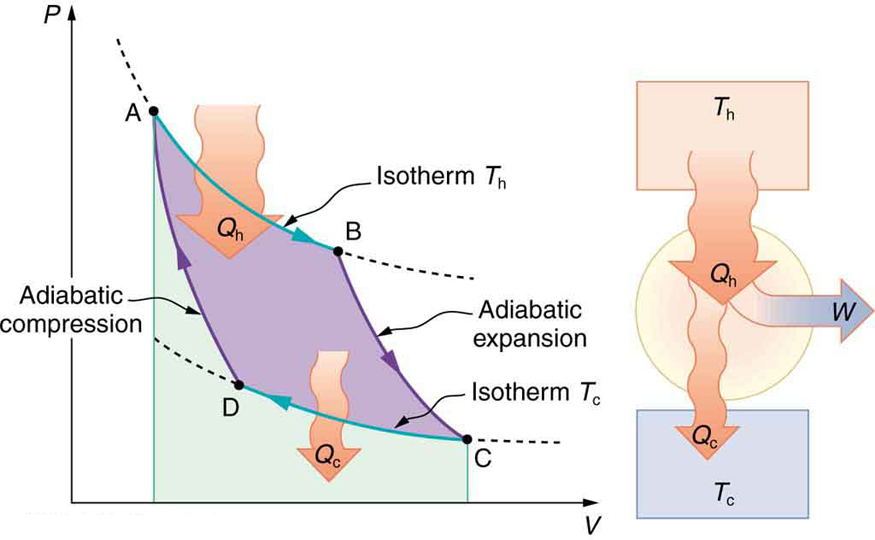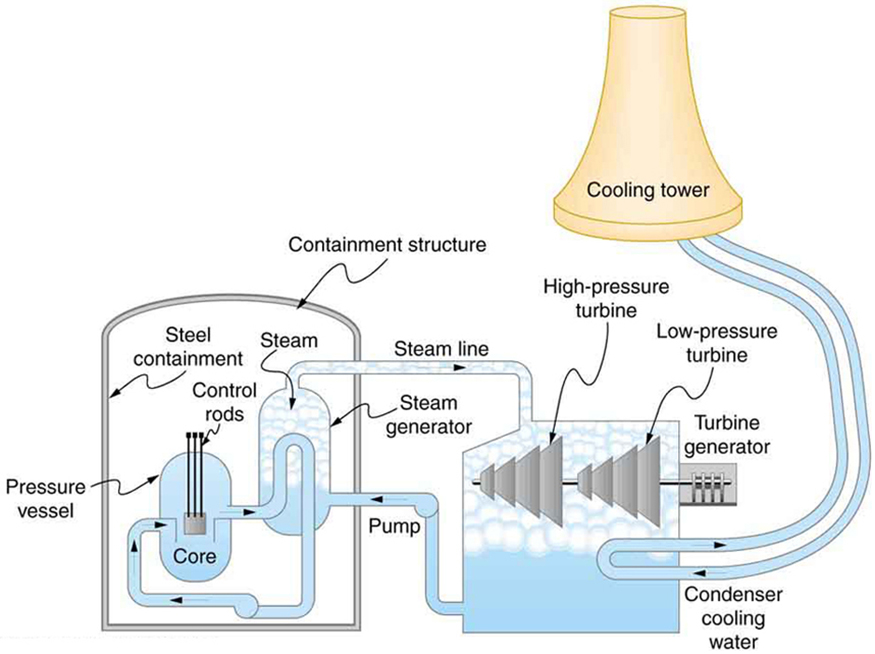# 15.4 Carnot’s perfect heat engine: the second law of thermodynamics  (Page 2/7)

 Page 2 / 7

Carnot’s interesting result implies that 100% efficiency would be possible only if ${T}_{\text{c}}=0 K$ —that is, only if the cold reservoir were at absolute zero, a practical and theoretical impossibility. But the physical implication is this—the only way to have all heat transfer go into doing work is to remove all thermal energy, and this requires a cold reservoir at absolute zero.

It is also apparent that the greatest efficiencies are obtained when the ratio ${T}_{\text{c}}/{T}_{\text{h}}$ is as small as possible. Just as discussed for the Otto cycle in the previous section, this means that efficiency is greatest for the highest possible temperature of the hot reservoir and lowest possible temperature of the cold reservoir. (This setup increases the area inside the closed loop on the $\text{PV}$ diagram; also, it seems reasonable that the greater the temperature difference, the easier it is to divert the heat transfer to work.) The actual reservoir temperatures of a heat engine are usually related to the type of heat source and the temperature of the environment into which heat transfer occurs. Consider the following example.PV size 12{ ital "PV"} {} diagram for a Carnot cycle, employing only reversible isothermal and adiabatic processes. Heat transfer Q h size 12{Q rSub { size 8{h} } } {} occurs into the working substance during the isothermal path AB, which takes place at constant temperature T h size 12{T rSub { size 8{h} } } {} . Heat transfer Q c size 12{Q rSub { size 8{c} } } {} occurs out of the working substance during the isothermal path CD, which takes place at constant temperature T c size 12{T rSub { size 8{c} } } {} . The net work output W size 12{W} {} equals the area inside the path ABCDA. Also shown is a schematic of a Carnot engine operating between hot and cold reservoirs at temperatures T h size 12{T rSub { size 8{h} } } {} and T c size 12{T rSub { size 8{c} } } {} . Any heat engine using reversible processes and operating between these two temperatures will have the same maximum efficiency as the Carnot engine.

## Maximum theoretical efficiency for a nuclear reactor

A nuclear power reactor has pressurized water at $\text{300}\text{º}\text{C}$ . (Higher temperatures are theoretically possible but practically not, due to limitations with materials used in the reactor.) Heat transfer from this water is a complex process (see [link] ). Steam, produced in the steam generator, is used to drive the turbine-generators. Eventually the steam is condensed to water at $\text{27}\text{º}\text{C}$ and then heated again to start the cycle over. Calculate the maximum theoretical efficiency for a heat engine operating between these two temperatures.Schematic diagram of a pressurized water nuclear reactor and the steam turbines that convert work into electrical energy. Heat exchange is used to generate steam, in part to avoid contamination of the generators with radioactivity. Two turbines are used because this is less expensive than operating a single generator that produces the same amount of electrical energy. The steam is condensed to liquid before being returned to the heat exchanger, to keep exit steam pressure low and aid the flow of steam through the turbines (equivalent to using a lower-temperature cold reservoir). The considerable energy associated with condensation must be dissipated into the local environment; in this example, a cooling tower is used so there is no direct heat transfer to an aquatic environment. (Note that the water going to the cooling tower does not come into contact with the steam flowing over the turbines.)

what is physics
what are the basic of physics
faith
tree physical properties of heat
tree is a type of organism that grows very tall and have a wood trunk and branches with leaves... how is that related to heat? what did you smoke man?
what are the uses of dimensional analysis
Dimensional Analysis. The study of relationships between physical quantities with the help of their dimensions and units of measurements is called dimensional analysis. We use dimensional analysis in order to convert a unit from one form to another.
Emmanuel
meaning of OE and making of the subscript nc
Negash
kinetic functional force
what is a principal wave?
A wave the movement of particles on rest position transferring energy from one place to another
Gabche
not wave. i need to know principal wave or waves.
Haider
principle wave is a superposition of wave when two or more waves meet at a point , whose amplitude is the algebraic sum of the amplitude of the waves
kindly define principal wave not principle wave (principle of super position) if u can understand my question
Haider
what is a model?
hi
Muhanned
why are electros emitted only when the frequency of the incident radiation is greater than a certain value
b/c u have to know that for emission of electron need specific amount of energy which are gain by electron for emission . if incident rays have that amount of energy electron can be emitted, otherwise no way.
Nazir
Nazir
what is ohm's law
states that electric current in a given metallic conductor is directly proportional to the potential difference applied between its end, provided that the temperature of the conductor and other physical factors such as length and cross-sectional area remains constant. mathematically V=IR
ANIEFIOK
hi
Gundala
A body travelling at a velocity of 30ms^-1 in a straight line is brought to rest by application of brakes. if it covers a distance of 100m during this period, find the retardation.
just use v^2-u^2=2as
Gundala
how often does electrolyte emits?
alhassan
just use +€^3.7°√π%-4¢•∆¥%
v^2-u^2=2as v=0,u=30,s=100 -30^2=2a*100 -900=200a a=-900/200 a=-4.5m/s^2
akinyemi
what's acceleration
The change in position of an object with respect to time
Mfizi
Acceleration is velocity all over time
Pamilerin
hi
Stephen
It's not It's the change of velocity relative to time
Laura
Velocity is the change of position relative to time
Laura
acceleration it is the rate of change in velocity with time
Stephen
acceleration is change in velocity per rate of time
Noara
what is ohm's law
Stephen
Ohm's law is related to resistance by which volatge is the multiplication of current and resistance ( U=RI)
Laura
acceleration is the rate of change. of displacement with time.
the rate of change of velocity is called acceleration
Asma
how i don understand
how do I access the Multiple Choice Questions? the button never works and the essay one doesn't either
How do you determine the magnitude of force
mass × acceleration OR Work done ÷ distance
SeemaByByBy John GabrieliBy OpenStaxBy Madison ChristianBy Katy PrattBy John GabrieliBy OpenStaxBy Jonathan LongByBy Richley CrapoBy JavaChamp Team